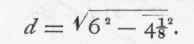Let ABC (Fig. 286) be the given triangle, and CE a perpendicular let fall upon A B, the base. Let the several lines of the figure be represented by the symbols a, b, c, d, g, and f as shown. Then, since A EC and BEC are right-angied triangles, we have (Art. 416) the following two equations, and, by subtracting one from the other, the third -Fig. 286.

f3 + d2 = a2 g2 + d2 = b2,

f2-g2=a2-b2 Then (Art. 414), by substitution, we have -

(f+g) (f-g) = (a+b) (a-b)

By division we obtain -

f- g = (a+b) (a-b)

f+g

According to Art. 431, equation (128.), we have -

g = f+g/2 - f-g/2

In this expression let the value of f - g, as above, be. substituted, then we will have -

g = f+g/2 - (a+b) (a-b)/2 (f+g).

Multiply the first fraction by (f + g), then join the two fractions, when we will have -

g = (f + g)2-(a + b)(a-b)/2 (f+g).

The lines f and g, in the figure, together equal the line c; therefore, by substitution -

g= c2- (a+b) (a-b)/2c (129.)

This is the value of the line g.

It may be expressed in words, thus: The shorter of the two parts into which the base of a triangle is divided by a perpendicular let fall from the apex upon the base, equals the quotient arising from a division by twice the base, of the difference between the square of the base and the product of the sum and difference of the two inclined lines.

As an example to show the application of this rule, let a = 9, b = 6, and c = 12; then equation (129.) becomes -

g = 122 (9+6) (9-6)/2 x12,

2 X 12

g = 144 - 15x3/24

g = 99/24 = 4 1/8.

Now, to obtain the length of d, the perpendicular, by the figure, we have -

d2 = b2-g2 -and, extracting the square root -(130.)

or, in words: The altitude of a triangle equals the square root of the difference of the squares of one of the inclined sides and its base.

As an example, take the same dimensions as before, then equation (130.) becomes -The square of 6 = 36. " " " 4* = 17.015625 62. 41/82 = 18..984375,

the square root of which is 4.44234; therefore -This may be tested by applying the rule to the other inclined side and its base -

c = 12 g= 4 1/8

f= 7 7/8

Then,92 = 81. 7 7/8 2= 62.015625

92.7 7/82= 18.984375.

The same result as before, producing for its square root the same, 4.44234, the value of d; therefore -433. - Triton: Radius of Circumscribed and Inscribed Circles: Area. - Let ABC (Fig. 287) be a given trigon or triangle with its circumscribed and inscribed circles. Draw the lines A D F, D B and D C

The three triangles, A B D, A CD, and B D C, have their apexes converging at D, and form there the three angles,

A D B, AD C, and B D C. These three angles together form four right angles (Art. 335), and each of them, therefore, equals 4/3 of a right angle.Fig. 287.

The angles of the triangle BDC together equal two right angles (Art. 345). As above, the angle BDC equals 4/3 of a right angle, hence 2 - 4/3 = 6-4/3 = 2/3 of a right angle, equals the sum of the two remaining angles at B and C. The triangle BDC is isoceles (Art. 338); for the two sides BD and D C, being radii, are equal; therefore the two angles at the base B and C are equal, and as their sum, as above, equals 2/3 of a right angle, therefore each angle equals 1/8 of a right angle. Draw the two lines FC and FB. Now, because D C and DF are radii, they are equal, hence DFC is an isoceles triangle.

It was before shown that the angle B D C equals 4/3 of a right angle; now, since the diameter A F bisects the chord B C, the angles B D E and E DC are equal, and each equals the half of the angle BD C or, 1/2 of 4/3 of a right angle equals 2/8 of a right angle. Deducting this from two right angles (the sum of the three angles of the triangle), or 2 - 2/3 = 1 1/3 = 4/3 of a right angle equals the sum of the angles at F and C; hence each equals the half of 4/3, or 2/3 of a right angle; therefore the triangle DFC is equilateral. The triangles DBF and DFC are equal. The angles B D C and BFC are equal; the line BC is perpendicular to DF and bisects it, making DE and EF equal; hence DE equals half DF, or DB, radii of the circumscribing circle. Therefore, putting R to represent B D, the radius of the circumscribing circle, and b = B C, a side of the triangle A B C, by Art. 416, we have -

BD2 = BE2+ DE2,

R2=(b/2)2+(R/2)2

Transferring and reducing -

R2 - (R/2)2 = (b/2)2,

R2 - R2/4 = b2/4,

R2 (1-1/4) = 1/4b2,

3/4 R2 = 1/4b2

R2 = 4/3 x 1/4b2 = 1/3b2 = b2/3,(131.)

Or, The Radius of the circumscribing circle of a regular trigon or equilateral triangle, equals a side of the triangle divided by the square root of 3.

By reference to Fig. 287 it will be observed, as was above shown, that D E = E F=DF/2 = BD/2 or, D E, the radius of the inscribed circle, equals half the radius of the circumscribed circle; or, again, dividing equation (131.) by 2, we have - -